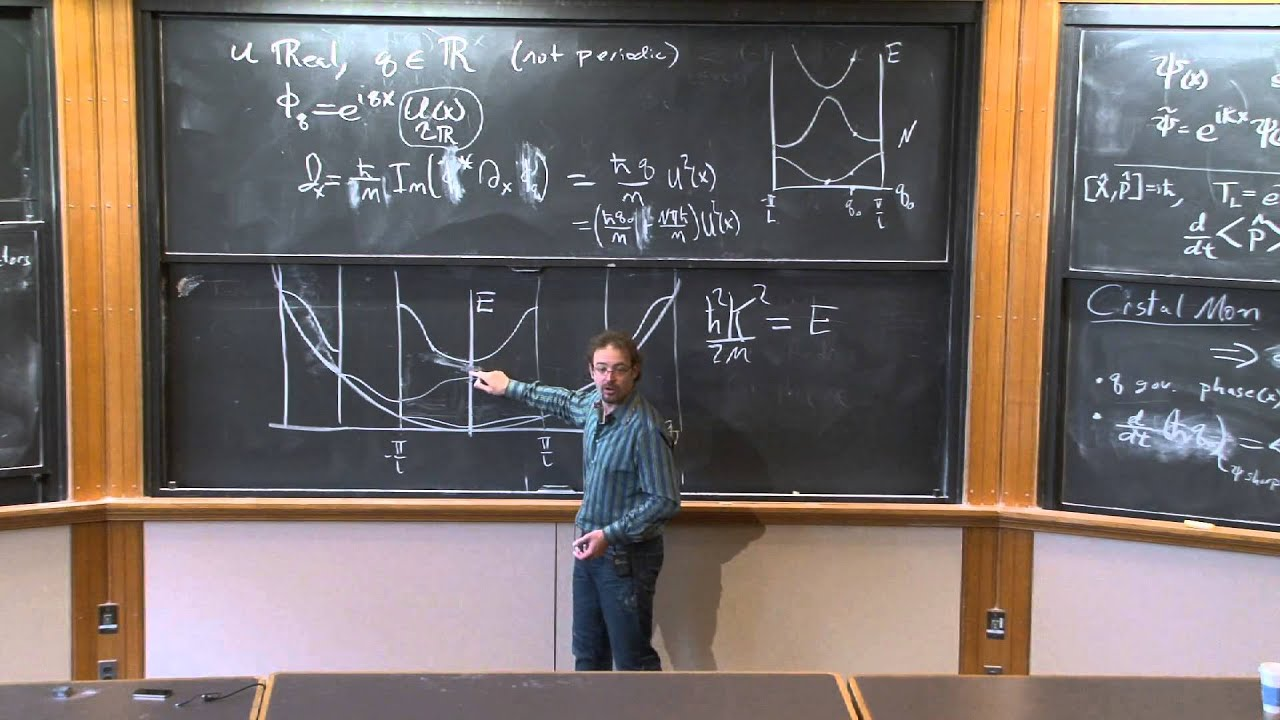MIT OpenCourseWare

# Quantum Physics I

This course covers the experimental basis of quantum physics. Topics include: photoelectric effect, Compton scattering, photons, Franck-Hertz experiment, the Bohr atom, electron diffraction, de Broglie waves, and the wave-particle duality of matter and light. Introduction to wave mechanics: Schrodinger's equation, wave functions, wave packets, probability amplitudes, stationary states, the Heisenberg uncertainty principle, and zero-point energies. Solutions to Schrodinger's equation in one dimension: transmission and reflection at a barrier, barrier penetration, potential wells, the simple harmonic oscillator. Schrodinger's equation in three dimensions: central potentials and introduction to hydrogenic systems. (from ocw.mit.edu )

Instructor

Physics
2014

#### Lecture 22 - Metals, Insulators, and Semiconductors